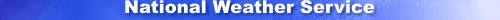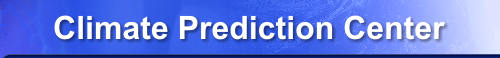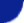Site Map News Organization SearchHOME > Monitoring_and_Data > Oceanic and Atmospheric Data > Reanalysis: Atmospheric Data > wgrib2 -if_n

Introduction

The -if_n option is an -if for a range of inventory numbers. The -if_n option uses the same syntax as the "for_n" option. If you want to operate on inventory records 10 to 20, you would use the parameter 10:20. If you want to operate on all the even inventory records from 10 to 20, you would use 10:20:2. The restrictions are the start value must be less than the ending value and the step has to be greater than zero.

The -if_n option is similar to the -for_n option in that they both select a range of invetory records. The difference is that the -for_n option selects the range of records that wgrib2 will process. With the the -if_n option, all the records will be processed but only the additional processing within the -if block will be only done for the selected records. The -for_n option can be much faster if the field has to be decoded. With the -for_n option, only the selected records need to be docoded whereas all the records would be decoded when using the -if_n option. The command line, however, can have multiple -if_n options.

Usage

-if_n I:J:K        same as for n = I to J by K
-if_n I:J          same as for n = I to J by 1
-if_n I::K         same as for n = I to MAX_INTEGER by K
-if_n I            same as for n = I to MAX_INTEGER by 1

Example

\$ wgrib2 file.grb2  -if_n 4:5 -s -fi
4:13335:d=2008120200:RH:750 mb:anl:
5:17098:d=2008120200:TMP:2743 m above mean sea level:anl: